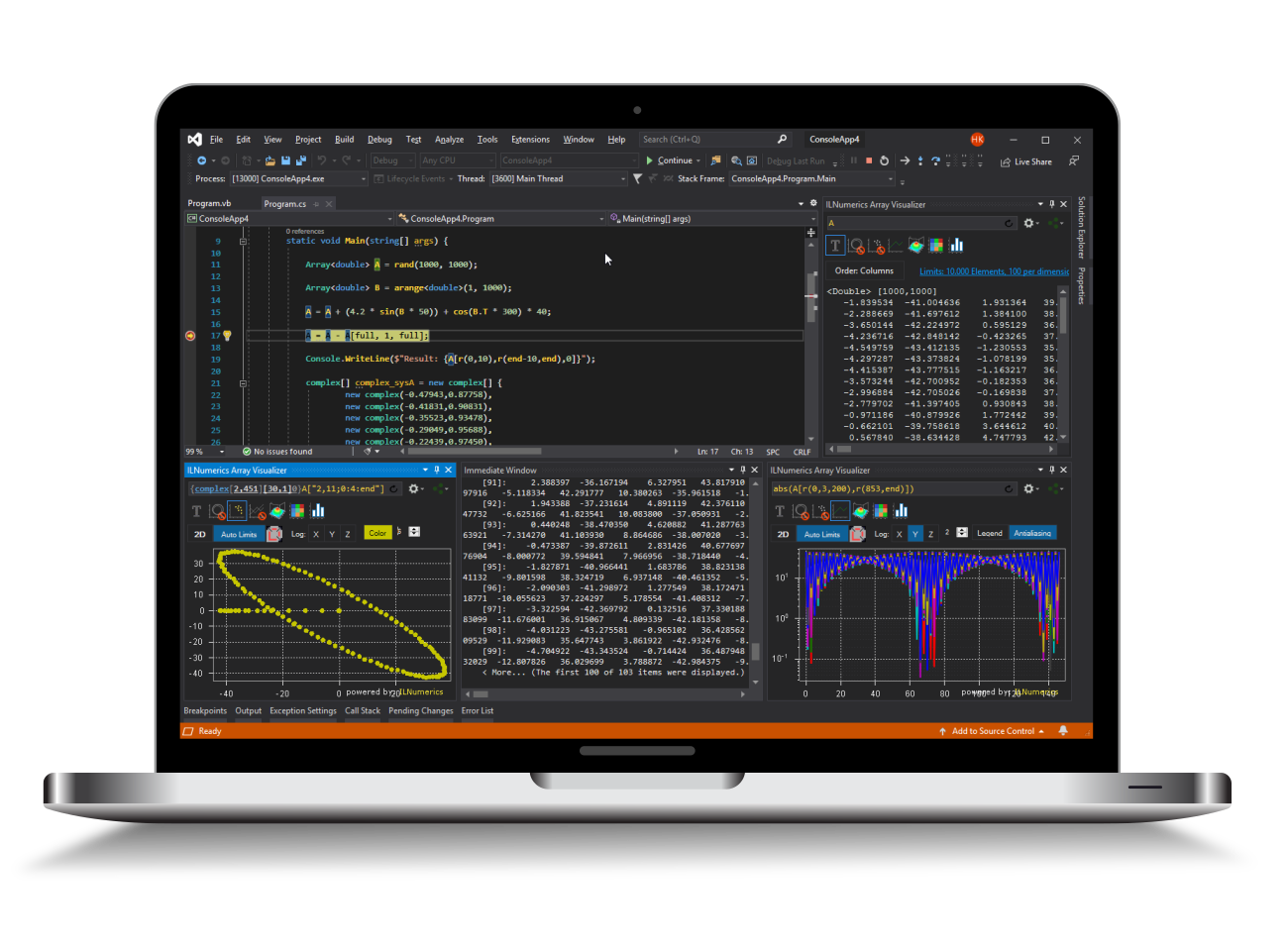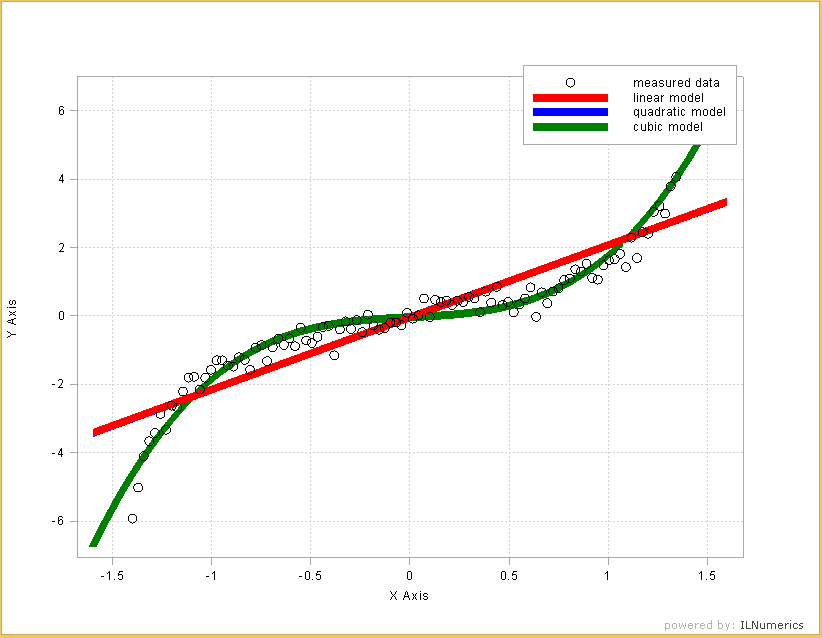Industrial Data Science
in C# and .NET:
Simple. Fast. Reliable.ILNumerics - Technical Computing

Modern High Performance Tools for Technical

Computing and Visualization in Industry and Science

tgt

# Polynomial Ridge Regression in .NET (C# and Visual Basic)The MachineLearning.ridge_regression method fits a polynomial model to the input data. The degree of the polynom is controlled by the user. The model builds a linear combination of polynomial terms based on the measurement data according to the specified degree. A cubic model, therefore contains $a_1 x + a_2 x^2 + a_3 x^3$ terms and computes the linear weights $a_1$,$a_2$, and $a_3$ which best models the input data.

After the model is created, it can be used efficiently in order to predict values for arbitrary new data. Therefore the model object contains all necessary precomputed structures, learned from the measurement data. The model structure returned from MachineLearning.ridge_regression should be used inside a using block and is disposed after the block was left.

As for all regression problems, the model selection is of major importance. From the above example can be seen that both, the linear as well as the quadratic model are no good fits for our data. Since there is no quadratic component in the data, the quadratic model comes out with a linear term only - and gives the exact same result as the linear model. Only the cubic model is able to fit the data sufficiently well in the observed range.